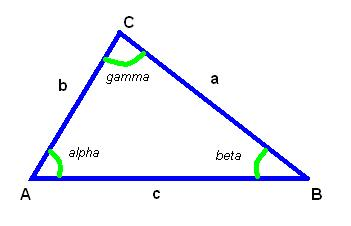homechevron_rightStudychevron_rightMathchevron_rightGeometry

# Lengths of triangle sides given one side and two angles

This online calculator determines lengths of triangle sides given one side and two anglesWell, there are miriad different ways to do math with triangle. I guess it is because triangle is the fundamental shape in geometry. Now this calculator is for those who wanted to determine lengths of triangle sides given one side and two angles.

It is actually simple, you just need to use law of sines, which looks like this:

That's it.#### Lengths of triangle sides given one side and two angles

°
°
Digits after the decimal point: 2
Side A

Side B

#### Similar calculatorsPLANETCALC, Lengths of triangle sides given one side and two angles